Last updated on August 03, 2019

We use if statements in our everyday life all the time – even if our everyday life is not written in Python. If the light is green then I’ll cross the road; otherwise I’ll wait. If the sun is up then I’ll get out of bed; otherwise I’ll go back to sleep. Okay, maybe it’s not this direct, but when we take actions based on conditions, our brain does what a computer would do: evaluate the conditions and act upon the results. Well, a computer script doesn’t have a subconscious mind, so for practicing data science we have to understand how an if statement works and how we can apply it in Python!

Note: This is a hands-on tutorial. I highly recommend doing the coding part with me – and if you have time, solving the exercises at the end of the article! If you haven’t done so yet, please go through these articles first:

## Python if statements basics

The logic of an if statement is very easy.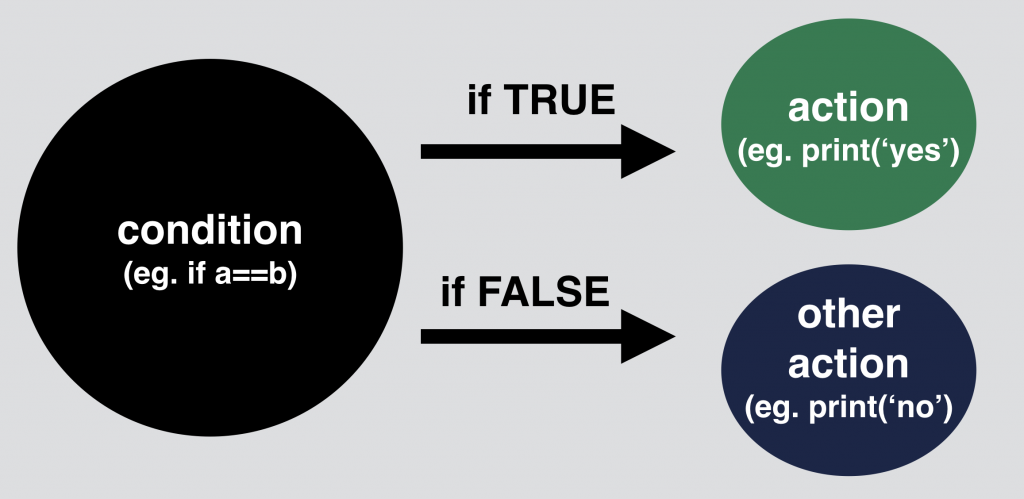Let’s say we have two values: `a = 10` and `b = 20`. We compare these two values: `a == b`. This comparison has either a `True` or a `False` output. (Test it in your Jupyter Notebook!)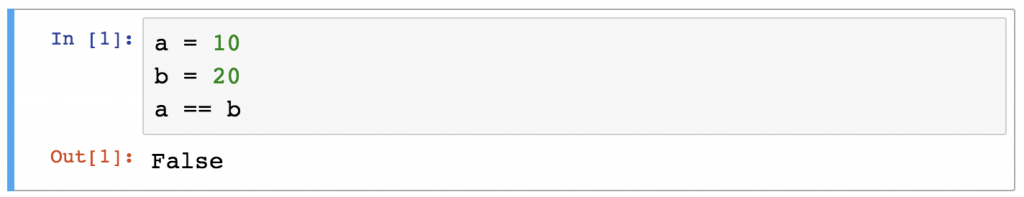We can go even further and set a condition: `if a == b` is `True` then we print `'yes'`. If it’s `False` then we print `'no'`. And that’s it, this is the logic of the Python if statements. Here’s the syntax:

```a = 10
b = 20
if a == b:
print('yes')
else:
print('no')
```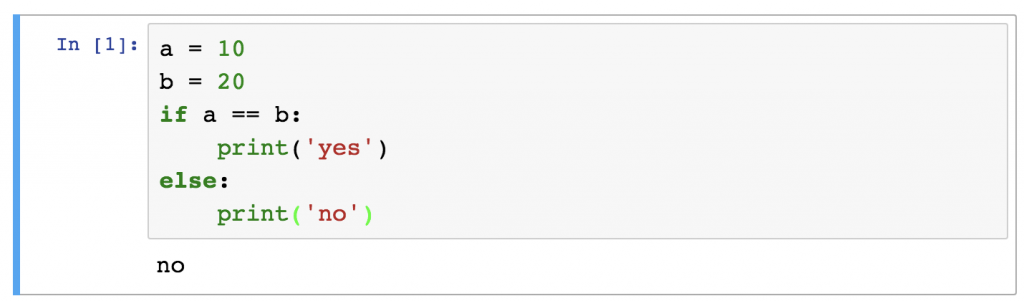Run this mini script in your Jupyter Notebook! The result will be (obviously): `no`.

Now, try the same – but set `b` to `10`!

```a = 10
b = 10
if a == b:
print('yes')
else:
print('no')
```

The returned message is `yes`.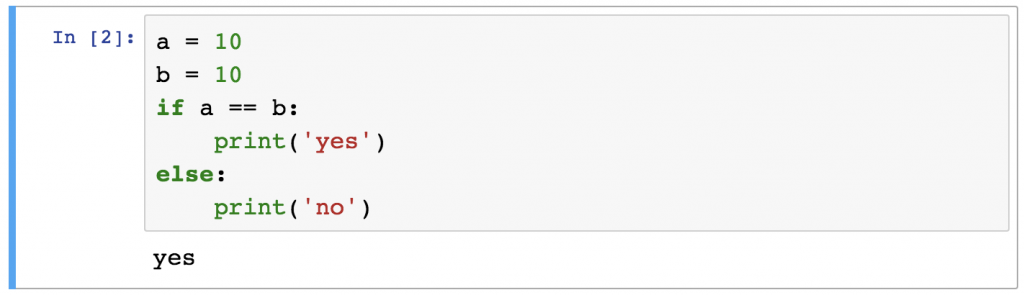## Python if statement syntax

Let’s take a look at the syntax, because it has pretty strict rules.
The basics are simple: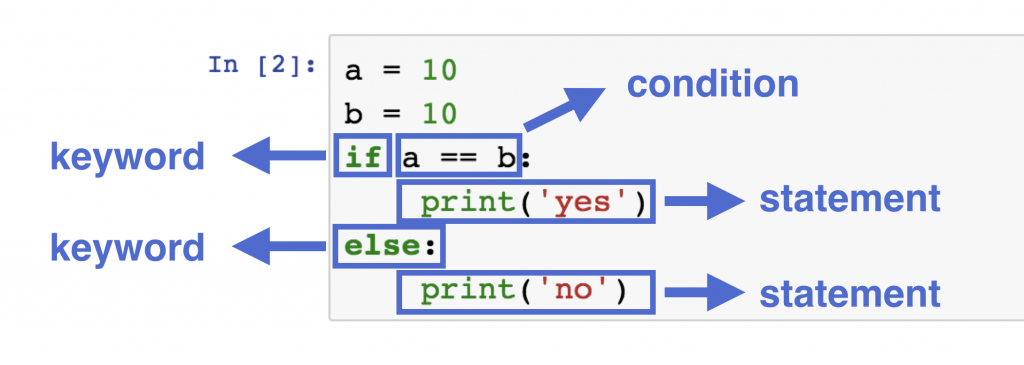You have:

1. an `if` keyword, then
2. a condition, then
3. a statement, then
4. an `else` keyword, then
5. another statement.

However, there are two things to watch out for:
1. Never miss the colons at the end of the if and else lines!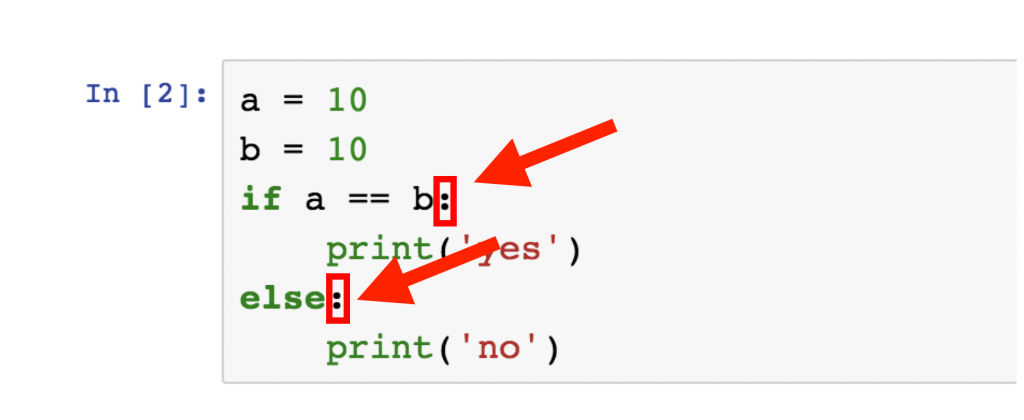2. And never miss the indentation at the beginning of the statement-lines!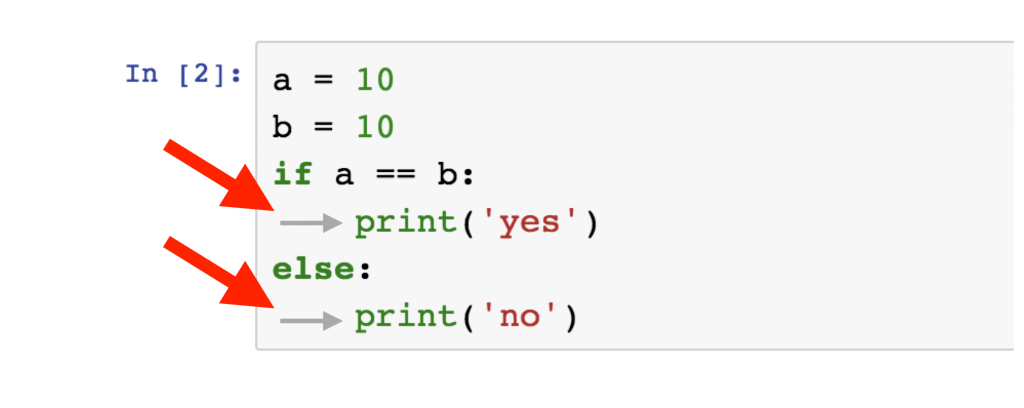If you miss any of the above two, an error message will be returned saying “invalid syntax” and your Python script will fail.

Note: if you are watching the Silicon Valley TV show, you might have heard about the “tabs vs spaces” debate. Here’s the hilarious scene:

What’s the real answer? Here’s what the original Style Guide for Python Code says:Pretty straight forward! 🙂
But, to be honest, I do use tabs because it’s much easier and – you know – “because I prefer… precision.”

## Python if statements – level 2

Now that you understand the basics, it’s time to make your conditions more complex – by using arithmetic, comparison and logical operators. (Note: if the word “operators” does not ring any bells, you might want to check out this article first: Python for Data Science – Tutorial for Beginners #1 – Python Basics.)

Here’s a quick example:

```a = 10
b = 20
c = 30
if (a + b) / c == 1 and c - b - a == 0:
print('yes')
else:
print('no')
```

This script will return `yes`, since both of the conditions, `(a + b) / c == 1` and `c - b - a == 0` are actually `True` and the logical operator between them was: `and`.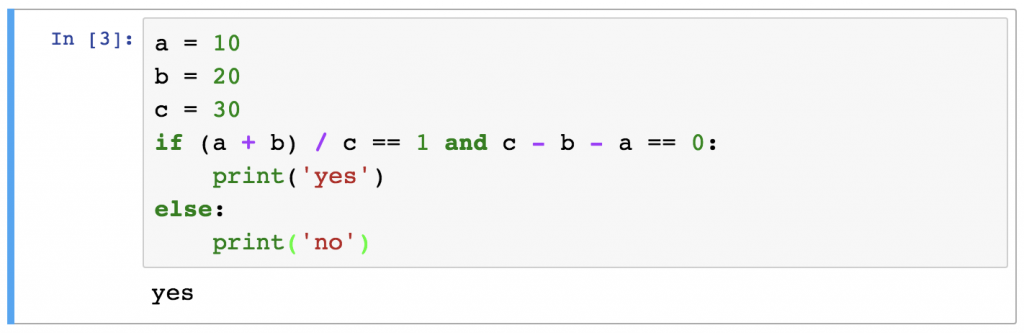Of course, you can make this even more complex if you want, but the point is: having multiple operators in an if statement is absolutely possible – in fact, it’s pretty common in real life scenarios!

## Python if statements – level 3

You can take it to the next level again, by using the `elif` keyword (which is a short form of the “else if” phrase) to create condition-sequences. “Condition-sequence” sounds fancy but what really happens here is just adding an if statement into an if statement: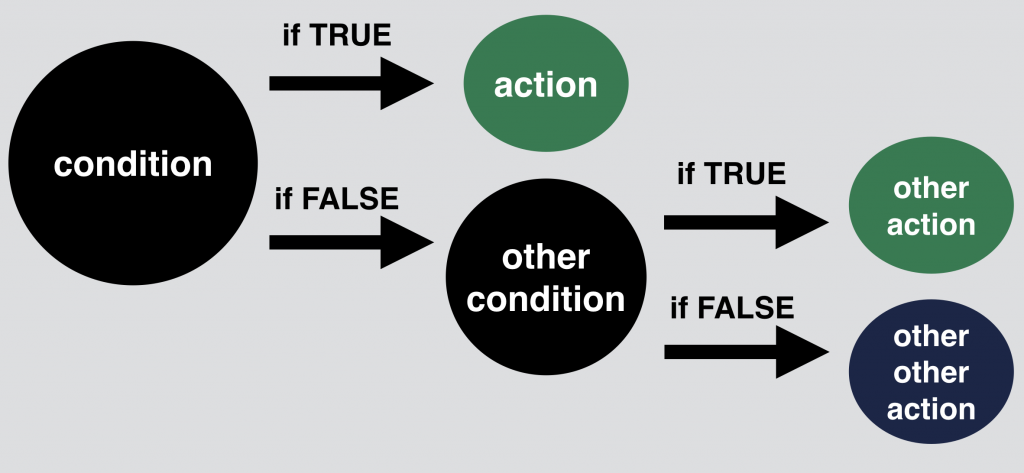Another example:

```a = 10
b = 11
c = 10
if a == b:
print('first condition is true')
elif a == c:
print('second condition is true')
else:
print('nothing is true. existence is pain.')
```

Sure enough the result will be `"second condition is true"`.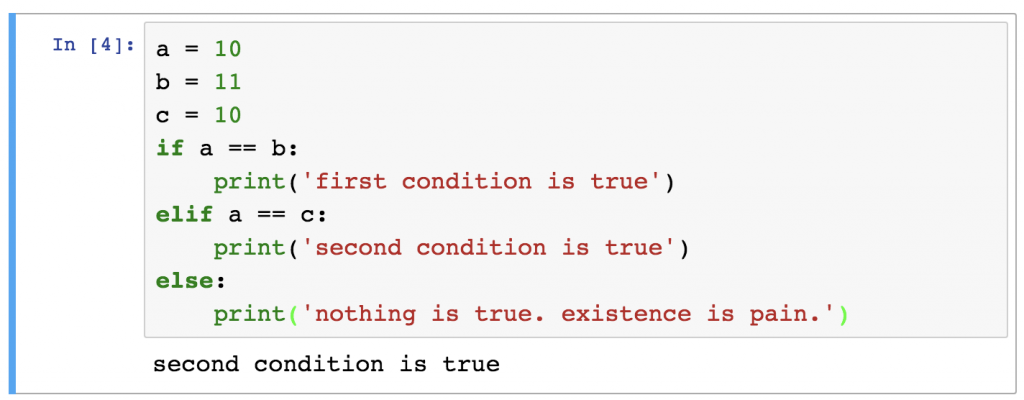You can do this infinite times, and build up a huge if-elif-elif-…-elif-else sequence if you want!

Aaand… This was more or less everything you have to know about Python if statements. It’s time to:

## Test yourself!

Here’s a random integer: `918652728452151`.

• Is it divisible by 17?
• Does it have more than 12 digits?

If both of these conditions are true, then I want to print “`super17`“.

And if either of the conditions are false, then I’d like to run a second test on it:

• Is it divisible by 13?
• Does it have more than 10 digits?

If both of these two new conditions are true, then I want to print “`awesome13`“.

And if the original number is not classified as “`super17`” nor “`awesome13`“, then I’ll just print: “`meh, this is just an average random number`“.

So: is `918652728452151` a super17, an awesome13 or just an average random number?

## The solution

`918652728452151` is a `super17` number!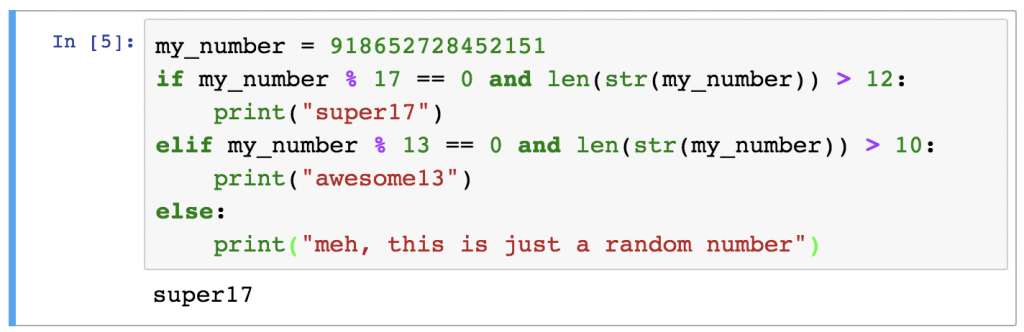Take a look at the script:

```my_number = 918652728452151
if my_number % 17 == 0 and len(str(my_number)) > 12:
print("super17")
elif my_number % 13 == 0 and len(str(my_number)) > 10:
print("awesome13")
else:
print("meh, this is just a random number")
```

On the first row, I’ve stored `918652728452151` into a variable so I don’t have to type it again and my script will be much nicer too: `my_number = 918652728452151`

Then, I set my if-elif-else condition sequence.
On the if line I wanted to specify two conditions. The first one is that the `my_number` variable is divisible by 17. This was the `my_number % 17 == 0` part. To be more accurate, this code means that the remainder from the division by 17 equals zero. The other half of the condition required counting the number of digits in `my_number`. Since – by the limitation of Python – you can’t count the number of digits in an integer, I had to turn my_number into a string with a `str()` function and then use the `len()` function on this string to get the number of characters. That was the `len(str(my_number))`.

It seems that both of my original conditions were true since I got back the `super17` on my screen. But if it weren’t then the next `elif` line would have done the same thing we have done in the if line, only it would have checked the divisibility by 13 (and not 17) and the number of digits should have been greater than 10 (and not 12.)

If that were not true either, my else statement would have been run to `print("meh, this is just a random number")`

That’s the solution! Wasn’t too difficult, was it?

## Summary

If statements are widely used in every programming language. Now you know how to use them too! The logic of it is super clear and on top of that, in Python, the syntax is even fully understandable by simply speaking in English…

Anyway. This was my introduction into Python If Statements. Next time we will continue with Python for loops!

Cheers,
Tomi Mester

1.#### Sri

I must say, the way you explain stuff is much simple compared to many online courses. I learnt so much from this blog and I’m waiting for 1-month online course.

I ditched pricey Udacity, CodeAcademy etc(on coding part) and learning DataScience in the order you recommended – SQL, Python, Bash from Data36. So just wanted to quickly check, how many more chapters will you be covering in Python and at what pace?

Thanks

•#### Tomi Mester

hi Sri,

thanks a lot! I’m really glad you like my tutorials!
As of Python basics, I’m planning 3 more episodes, one of them is already online! : )
And I’ll write an advanced Python series as well later this year!

Cheers,
Tomi

2.#### Nisha

Just realized, all functions & operators are case-sensitive (e.g. it should be and not AND) unlike variable (you can define variable by A but in argument, you can use a)

•#### Tomi Mester

Yepp, that’s true, I’ll write a separate article about Python best practices and syntax questions!
Tomi

3.#### john schlafly

Hey Tomi,

FYI your fake door is still up on Lesson 3, even tho there is a Lesson 4 now. I found it interesting tho, have never heard of those before 🙂

•#### Tomi Mester

Ha! Thanks!

4.#### Ivan

Hey Tomi,
I find no link to the next episode at the end of this one.
Thanks!

•#### Tomi Mester

Thanks Ivan! I’ve just fixed this!

5.#### VJ

Hi Tomi,

Thank you for explaining in such a simple manner by breaking compound concept into smaller understandable chunks each with suitable example!!

•#### Tomi Mester

cheers!

6.#### Vinay Suresh

I found an interesting alternative to the solution

```x = 918652728452151
a = abs(x/17)
b = abs(x/13)
if (round(a) - a) == 0 and len(str(x)) > 12:
print ('super17')
elif (round(b) - b) == 0 and len(str(x)) > 10:
print ('awesome13')
else:
print ('meh, this is just an average random number')
```

I didnt know about % as I skipped that section. But I thought of sharing my solution.
Brilliant tutorials. Love your method of approach. It is quite easy to grasp your explanations.

•#### Tomi Mester

Thanks a lot for the kind words –» and especially for the alternative solution!
Enjoy the rest of the blog!

Tomi